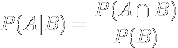# Conditional probability

A Visual explanation by Victor Powell for Setosa

A conditional probability is the probability of an event, given some other event has already occurred. In the below example, there are two possible events that can occur. A ball falling could either hit the red shelf (we'll call this event A) or hit the blue shelf (we'll call this event B) or both.

If we know the statistics of these events across the entire population and then were to be given a single ball and told "this ball hit the red shelf (event A), what's the probability it also hit the blue shelf (event B)?" we could answer this question by providing the conditional probability of B given that A occurred or P(B|A).= {{pOfA | number:3}} or {{pOfA * 100 | number:1}}%

= {{pOfB | number:3}} or {{pOfB * 100 | number:1}}%

= {{pOfAAndB | number:3}} or {{pOfAAndB * 100 | number:1}}%

P(B|A) = {{ pOfBGivenA | number:3}} or {{ pOfBGivenA * 100 | number:1}}%

If we have a ball and we know it hit the red shelf, there's a {{ pOfBGivenA * 100 | number:1}}% chance it also hit the blue shelf.

P(A|B) = {{ pOfAGivenB | number:3}} or {{ pOfAGivenB * 100 | number:1}}%

If we have a ball and we know it hit the blue shelf, there's a {{ pOfAGivenB * 100 | number:1}}% chance it also hit the red shelf.

actual

expected

count(A n !B): {{countA}} (balls that hit the red shelf but not the blue shelf)

count(B n !A): {{countB}} (balls that hit the blue shelf but not the red shelf)

count(A n B): {{countAB}} (balls that hit both the red shelf and the blue shelf)

count(!A n !B): {{countNone}} (balls that did not hit the red nor blue shelf)